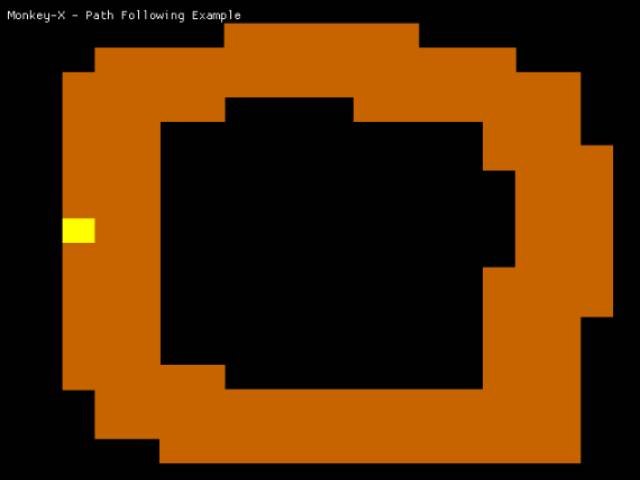-=+=- -=+=- -=+=- -=+=- -=+=- -=+=- -=+=- -=+=- -=+=- -=+=- -=+=- -=+=- -=+=- -=+=- -=+=- -=+=- -=+=- -=+=- -=+=- -=+=- -=+=- -=+=- -=+=- -=+=- -=+=- -=+=- -=+=- -=+=- -=+=- -=+=- (c) WidthPadding Industries 1987 0|407|0 -=+=- -=+=- -=+=- -=+=- -=+=- -=+=- -=+=- -=+=- -=+=- -=+=- -=+=- -=+=- -=+=- -=+=- -=+=- -=+=- -=+=- -=+=- -=+=- -=+=- -=+=- -=+=- -=+=- -=+=- -=+=- -=+=- -=+=- -=+=- -=+=- -=+=-
SoCoder -> Snippet Home -> AI and Movement

PakzCreated : 20 February 2017

Language : Monkey

### Path Following

From the book 'ai for game developers'Here the html 5 page :
cromdesi.home.xs4all.nl/html5/pathfollowing/MonkeyGame.html

Another thing I started to understand from the book 'ai for game developers' Path following.
Here a entity is put on a road and he follows the road. This is done with 'weight' Where the forward direction has a greater weight and the other directions a lesser weight.

It does not seem to work well on every kind of road though.

The book explained more about how and what it can be used for. My main interest was the use in rpg type games.